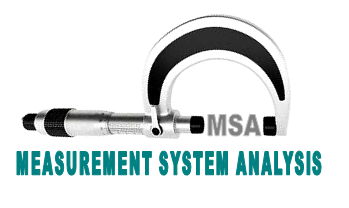## Measurement system analysis (MSA) – Analysis of measuring devicesMSA (Measurement System Analysis) aim is to ensure that measurement systems used in organization are working within acceptable range of measurement uncertainty. To measuring devices to be studies are possible if t he person are well training for study and analysis for calculations of the results as systematically.

### How to Proceed MSA(Measurement System Analysis)?

1. #### For variable data measurement gauges/instruments measuring repetitive data ‘Gauge R & R’ shall be used as the method of calculating measurement uncertainty.

• Decide the instrument for which Gauge R & R is to be calculated.
• Select 10 different samples from a batch / lot, which being manufacture throughout production lot cycle.
• Select three different inspectors doing this stage inspection activity.
• Ask the inspectors to inspect the 10 pieces (specified parameters) & record the readings.
• Each inspector shall conduct 3 trials of inspection on the 10 sample pieces.
• Calculate average readings & range of trial averages for each inspector.
• Calculate difference of inspector’s averages, average range & within part range for the study.
• Calculate repeatability (equipment variation), reproducibility (appraiser variation), R & R, part variation, total variation, relevant percentages & ‘NDC’ (No. of distinct categories)
• Gauge R & R % shall be used as basis for accepting the gauge as below:
• R & R Below 10 % Measurement system is acceptable.
• R & R 10 % to 20 % Measurement system may be acceptable based upon importance of application, cost of gauge, cost of repair etc.
• R & R over 30 % Measurement system needs improvement.
• For a good measurement system NDC (Number of distinct categories) shall be minimum 5.
• Actions identified to improve measurement system shall be implemented & effect shall be verified.
• Gauge R & R studies shall be conducted whenever
• In case new type of measurement system is introduce.
• Improvements in current measurement system due to results of Gauge R & R studies.
• Every year irrespective of above 2 conditions.
1. #### Measurement uncertainty of attribute gauges can be calculate  using short method as are below:

• Choose the gauge to be studied.
• Select 10 samples from a production lot at different times.
• At least Select 2 different inspectors doing this stage inspection activity regularly.
• Ask the inspectors to take two trials on each sample & record the results. Calculate ‘kappa’ value.
• If ‘CAPA’ value is more than 0.6 the gauge shall be accepted. Otherwise the gauge shall be improved /reconditioned; if not possible it should be scrapped & replaced by new gauge.
• Gauge acceptance study as above shall be conducted minimum for 1 gauge of each type for every year.
1. #### BIAS of measuring device used for repetitive measurement of variable data can calculate as  are below:

• Select a known Instrument.
• Take min. 20 repetitive readings of same Instrument, every time adjusting the equipment to zero setting.
• Calculate bias as difference between average value of measurement and known Instrument.
• Linearity and Uniformity studies can  conduct for measurement systems are in use, to measure different spans of measurement.
• Select different Instruments over span of measurement of system.
• Take minimum 10 readings of each reference.
• Calculate Bias and Repeatability for each set of observations.
• Linearity is indicate by change in bias over span of measurement.
• Uniformity is indicate by change in repeat-ability over span of measurement.

Measurement system analysis’s result should be acceptance or rejection of the whole exercise that can be considering the devices / instruments are in acceptable level? or not?

### orbit

Orbit is a expert in solutions of industrial problems viz quality, production, planning, inspection etc. Orbit is writes about manufacturing's problems and its state solutions for quality lovers..